# Cantor dust

## fractal

last updated: 2005-01-07

George Cantor (1845-1918) constructed the Cantor dust.
The curve is a base motif fractal which uses a square segment as base. The motif is to divide the square into nine equal parts and let only the outer four remain: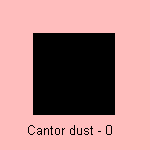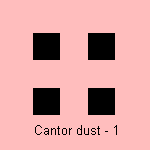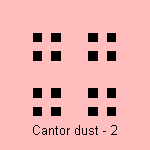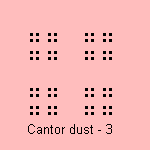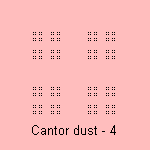The fractal dimension of the Cantor dust is equal to log4/log3, what is about 1.26186 1).

A variant on this curve is given by the box fractal: divide the square into 9 equal parts and let the five diagonal parts remain.
This curve is also named the anticross-stitch: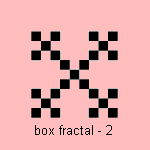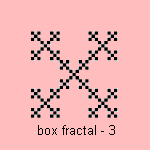The fractal dimension of the box fractal is equal to log5/log3, what is about 1.46497 2).

An alternative Cantor dust has as motif to divide the square into 16 equal parts and let only (arbitrary) four remain.
This makes a fractal with dimensions 1, you can imagine that in this way a square turns into a line 3).

Sometimes the Cantor dust name is given to the Cantor set.

notes

1) Fractal dimension = log N / log e, where N is the number of segments and e the magnification.
For the Cantor dust: N=4, e=3.

2) Fractal dimension = log N / log e, where N is the number of segments and e the magnification.
For the box fractal: N=5, e=3.

3) Fractal dimension = log N / log e, where N is the number of segments and e the magnification.
For the alternative Cantor dust: N=4, e=4.